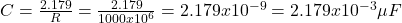## Now Ishani and John are ready to use what they know to build the turn indicator. They decide that the signal in their cars goes about once p

Question

Now Ishani and John are ready to use what they know to build the turn indicator. They decide that the signal in their cars goes about once per second. Assuming that they set the trigger to make the indicator go when charge has reached 1 − 1/e of its final value, and that it takes about the same amount of time to discharge as to charge, suggest values for R and C that could be used. (Assume that the capacitor reaches approximately its maximum value, discharges to 1 − 1/e of its maximum value over the course of 1 second, and then begins charging again.)’

in progress 0
3 months 2021-07-19T16:30:33+00:00 1 Answers 10 views 0

For a resistance (R) of 1000 MΩ and capacitance (C) of 2.179×10⁻³μF

Explanation:

The charge on the discharging capacitor is equal: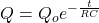If t = 1 s, then: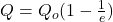Matching both equations: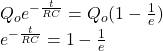If t = 1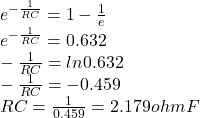The capacitance for a resistor of resistance of 1000 MΩ is equal: